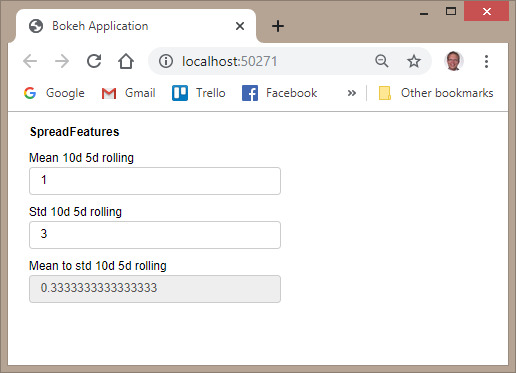# Is there a best practice way to create a param "property"?

I am trying to create something like a parameter `property` on `Parameterized` class. Because I would like be able to display and layout the `properties` in Panel via the `pn.Param` parameter.

As far as I can see this is not supported “out of the box” (?) so I’m creating the below workaround

``````import param

"""A class containing features of a spread"""

mean_10d_5d_rolling = param.Number()
std_10d_5d_rolling = param.Number()

mean_to_std_10d_5d_rolling = param.Number(None, constant=True)

@param.depends("mean_10d_5d_rolling", "std_10d_5d_rolling", watch=True)
def set_mean_to_std_10d_5d_rolling(self):
print("update")
with param.edit_constant(self):
if self.std_10d_5d_rolling:
self.mean_to_std_10d_5d_rolling = self.mean_10d_5d_rolling / self.std_10d_5d_rolling
else:
self.mean_to_std_10d_5d_rolling = None

``````

When I use IPython I see

``````In : %run scripts\issues\issue_param_constructor.py

In : features

In : features.set_mean_to_std_10d_5d_rolling()
update

In : features

In : features.std_10d_5d_rolling=3
update

In : features

In : features.std_10d_5d_rolling=3
update

In : features

In : import panel as pn

In : pn.Param(features).show()
``````I.e. the “setters” are not run when I construct the object. But only when I use the object after construction.

Is there a “param” way of specifying that events should be triggered during construction or do I need to override the parent constructor and implement this my self?

Is there a better way of achieving what I wan’t to do here?

This code achieves what I wan’t but I would still like to know if I’m approaching this right?

``````import param

"""A class containing features of a spread"""

mean_10d_5d_rolling = param.Number()
std_10d_5d_rolling = param.Number()

mean_to_std_10d_5d_rolling = param.Number(None, constant=True)

def __init__(self, **params):
super().__init__(**params)

self.set_mean_to_std_10d_5d_rolling()

@param.depends("mean_10d_5d_rolling", "std_10d_5d_rolling", watch=True)
def set_mean_to_std_10d_5d_rolling(self):
print("update")
with param.edit_constant(self):
if self.std_10d_5d_rolling:
self.mean_to_std_10d_5d_rolling = self.mean_10d_5d_rolling / self.std_10d_5d_rolling
else:
self.mean_to_std_10d_5d_rolling = None

``````In : %run scripts\issues\issue_param_constructor.py